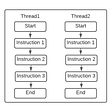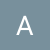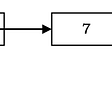# Target Sum

`Input: nums is [1, 1, 1, 1, 1], S is 3. Output: 5Explanation: -1+1+1+1+1 = 3+1-1+1+1+1 = 3+1+1-1+1+1 = 3+1+1+1-1+1 = 3+1+1+1+1-1 = 3There are 5 ways to assign + - to make the sum of nums be target 3.`
`/*package whatever //do not write package name here */import java.io.*;class Main {    // function that just calls dfs   // to calculate number of ways static int findWays(int[] nums, int S) {        return dfs(nums, S, 0, 0);    }      //dfs function    static int dfs(int[] nums, int S, int curr_sum, int index) {      // base case when we reaches the end of array      // and check whether the currSum is same asa      // required sum        if (index == nums.length) {          if(S==curr_sum) return 1;          else return 0;        }              // first adding the current index element       // to the currSum and calling dfs       //second subtracting current index element       // to the currSum and calling dfs      // adding both        return dfs(nums, S, curr_sum + nums[index], index + 1)           + dfs(nums, S, curr_sum - nums[index], index + 1);    }       // driver fucntion    public static void main (String[] args) {      int S = 3;      int[] arr = new int[]{1,2,3,4,5};      int answer =findWays(arr, S);        System.out.println("Number of ways are "+ answer);    }    }`
`/*package whatever //do not write package name here */import java.io.*;import java.util.*;class Main {    // function that just calls dfs   // to calculate number of ways static int findWays(int[] nums, int S) {      int sum=0;      for(int i=0 ; i<nums.length ; i++) sum+=nums[i];       int[][] memo = new int[nums.length + 1][2*sum+1];        for (int[] m: memo) {            Arrays.fill(m, Integer.MIN_VALUE);        }        return dfs(memo,nums, S, 0, 0, sum);    }      //dfs function    static int dfs(int[][] memo,int[] nums, int S, int curr_sum, int index, int sum) {      // base case when we reaches the end of array      // and check whether the currSum is same asa      // required sum        if (index == nums.length) {          if(S==curr_sum) return 1;          else return 0;        }// if we have current iteration in our       // memo table , we return that value        if (memo[index][curr_sum + sum] != Integer.MIN_VALUE) {            return memo[index][curr_sum + sum];        }               // first adding the current index element       // to the currSum and calling dfs       //second subtracting current index element       // to the currSum and calling dfs      // adding both and storing in memo             int ans =            dfs(memo, nums, index + 1, curr_sum + nums[index], S , sum) +            dfs(memo, nums, index + 1, curr_sum - nums[index], S, sum);        memo[index][curr_sum + sum] = ans;        return ans;                }       // driver fucntion    public static void main (String[] args) {      int S = 3;      int[] arr = new int[]{1,1,1,1,1};      int answer =findWays(arr, S);        System.out.println("Number of ways are "+ answer);    }    }`
`/*package whatever //do not write package name here */import java.io.*;class Main {    // function that just calls dfs   // to calculate number of ways static int knapSack(int[] nums, int S) {        int sum = 0;        for (int i: nums)           sum += i;      //if target + sum(nums) is not divisible by 2,      //we can return 0 because there is no solution      // also if sum is smaller than  S or negative of sum      // is greater than S functions return 0        if (sum < S || -sum > -S || (S + sum) % 2 == 1)            return 0;                int[] dp = new int[(S + sum) / 2 + 1];             // Since there is only 1 way to form a sum of        //0 using 0 nums        dp = 1;                for (int num: nums) {            for (int i = dp.length - 1; i >= num; i--) {              //number of ways to get sum i without num is the               //value in dp[last iteration of same column].              // number of ways to get sum I with num is the value in              // dp[i-num of last iteration]                dp[i] += dp[i - num];             }        }        // return the answer        return dp[dp.length - 1];    }       // driver fucntion    public static void main (String[] args) {      int S = 3;      int[] arr = new int[]{1,2,3,4,5};      int answer =knapSack(arr, S);        System.out.println("Number of ways are "+ answer);    }    }`

--

--

--

## More from Abhinay Gupta

Love podcasts or audiobooks? Learn on the go with our new app.

## Introduction to admin and applications in Django web framework## 7 Reasons Why You Need to Outsource a PM for Your Project## Kubernetes Service Mesh: Istio Edition## Flutter MobX Example Architecture## TRICK: Merge multiple CSV files into a single DataFrame with just one line of code!## How to Create a Flipbook for Offline Usage## Python Multi-Threading and the Office## 4-in-1 Priority First Search in Python: BFS, DFS, Prim’s, and Dijkstra’s algorithms## Google Foobar Challenge. What You Should Know## An Introduction to Linked Lists## LeetCode 2095- Delete the Middle Node of a Linked List